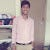Variance and Standard Deviation and Covariance.

Variance:

Variance is defined as how far the data is from its average value, in other words variance specifies or tells how the data is dispersed around its mean value.

So Variance is the ratio of sum of squared distance between the observed value…

Measure of Central Tendency

Firstly what is Central Tendency?

In Statistics Central Tendency is a single value that accurately summarizes the data or distribution of data. …## Krishnachaitanya

Aspiring to be a Data Scientist